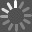One to Nine The Inner Life of Numbers Book - 2008

The first rule is unsurprising, but the second is highly unobvious: (a, b) + (c, d) = (a + c, b + d) (a, b) × (c, d) = (a × c − b × d, a × d + b × c). The natural numbers 1, 2, 3 … can be identified with the pairs (1, 0), (2, 0), (3, 0) … and for these, the new addition and multiplication coincides with what we had before. The same goes for the fractions and decimals: 1/2 is (1/2, 0) and π is (π, 0). It goes for negative numbers too: so (−1, 0) is the same as −1. But something new happens when the second element in the pair is used. Following the rules, (0, 1) × (0, 1) = (−1, 0). The pair (0, 1) behaves as a square root of minus one.
===

The K for a thousand, the third power of ten, is convenient because it is close to 1024, the tenth power of two. Less obvious is that the approximation 1024 ≈ 1000 is related to the problem of music, and this gives a starting point for both the harmonies and the cussedness of numbers.

jimg2000's rating:
[]
[]